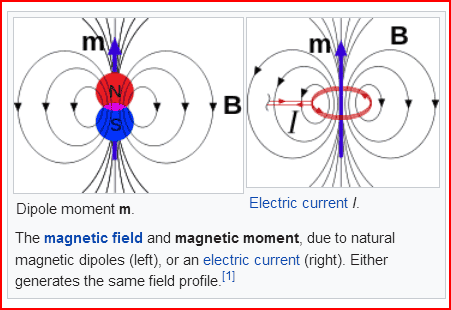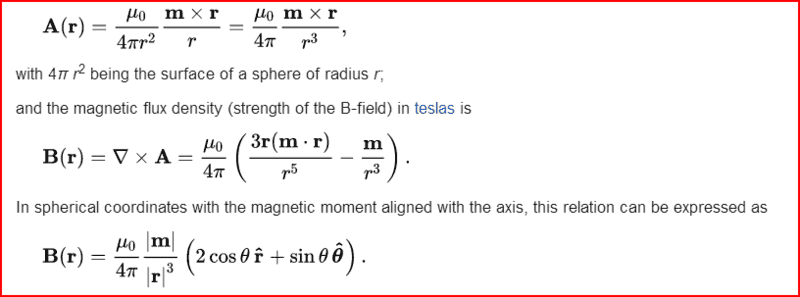# Radial dependence of the Sun's magnetic field

• I
• prodi
A magnetic dipole is a type of two-pole magnet with an axis that is not perpendicular to the direction of the magnetic field. The magnetic field lines cross at the dipole's center of mass. The dipole magnetic field is approximately half the strength of a magnetic field due to a single pole.f

#### prodi

I was solving some problems from a textbook and I found this formula for the radial dependence of the sun's magnetic field, ##B(r) \approx B_{\odot} \Big(\frac{R_{\odot}}{r}\Big)^{1/2}##, where ##B_\odot \approx 0.001T## is the magnetic field strength in the photosphere.
But how can this formula be derived and what principles are used for that?
I am thinking about assuming that magnetic flux is conserved, ##4\pi R_\odot^2 B_\odot=4 \pi r^2 B(r)##. Thus ##B(r)=B_\odot \Big(\frac{R_\odot}{r}\Big)^2##, but the exponent is 2 instead of 1/2.
Why am I wrong? Doesn't the magnetic flux conserve?

The sources for this magnetic field are currents that can be from different locations around the sun. The equation is apparently applicable at distances ## r ## from the center that are on the order of the radius of the sun. For this kind of close range behavior, you can not expect to see inverse square law type behavior. ## \\ ## Meanwhile, for magnetic fields, ## \nabla \cdot B=0 ## says that the magnetic lines of flux are conserved. For magnetic type sources, e.g. sources consisting of what can be approximated by something such as a cylindrical magnet with two poles, that doesn't necessarily mean that inverse square law behavior will occur. It simply means the flux lines for ## B ## are continuous as they go through the magnet and emerge from one end and return in the other. See https://www.google.com/imgres?imgur...hUKEwikmtrR74HdAhVCAqwKHZ5-Ar4Q9QEwAHoECAcQBg ## \\ ## And you might want to check the decimal place on your formula. A google shows the magnetic field of the sun near and around its surface is ## B \approx 1.0 ## gauss ## =0.0001 \, T ##.

•prodi
Thank you for the explanation.
Yes, you are right. It is ##0.0001 T##.
Can the inverse square law be used at a larger distance from the sun? Let's say ##r \approx 50R_\odot##.

Thank you for the explanation.
Yes, you are right. It is ##0.0001 T##.
Can the inverse square law be used at a larger distance from the sun? Let's say ##r \approx 50R_\odot##.
For the effect of the field from a single pole, such as the + pole of a permanent magnet, the inverse square law can apply, but when the effects of two poles (+ and -) are considered together, the result is often inverse cube. So the answer to that is no.

•prodi and berkeman
when the effects of two poles (+ and -) are considered together, the result is often inverse cube.
From the Wikipedia article on magnetic dipoles...

https://en.wikipedia.org/wiki/Magnetic_dipole#### Attachments

•